American Journal of Physics and Applications
Volume 4, Issue 1, January 2016, Pages: 1-4

Dispersion Relation of Waves in Hot Plasma Located in Rotating Electromagnetic Field

Albert Kazadi Mukenga Bantu*, Nyamu Molibi, Liyoko Mboyo, Alain Musongela Lubo, Philippe Badibanga Mudibu

Department of Physics, University of Kinshasa, Kinshasa, Democratic Republic of Congo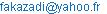(A. K. M. Bantu)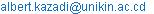(A. K. M. Bantu)

Albert Kazadi Mukenga Bantu, Nyamu Molibi, Liyoko Mboyo, Alain Musongela Lubo, Philippe Badibanga Mudibu. Dispersion Relation of Waves in Hot Plasma Located in Rotating Electromagnetic Field. American Journal of Physics and Applications. Vol. 4, No. 1, 2016, pp. 1-4. doi: 10.11648/j.ajpa.20160401.11

Abstract: The procedure used to obtain the expression of the dielectric tensor of cold plasma in a rotating electromagnetic field has been presented in our previous paper. We used this procedure to derivate the dielectric tensor for hot plasma in a rotating electromagnetic field. By means of the expression of dielectric tensor which expresses the linear response of plasma, we derived, discussed and compared the dispersion relation for waves in hot plasma with the one obtained for cold plasma located in a rotating electromagnetic field. This dispersion relation, which is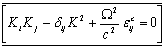, depends on three variables: wave's vector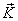, angular frequency Ω and temperature parameter Ta of particles kind ''a''. The super fix "c" means "hot" in this relation. We observed that more the temperature is higher, more is the electrical conductivity of plasma (weak is the resistivity of hot plasma). The study revealed that the dispersion relation has a temperature parameter in its exponential part. We observe also that: 1) when the temperature parameter Ta tends to zero, the exponential factor tends to unity.is the dispersion relation of cold plasma, where the super fix "f" means "cold". 2) the temperature parameter Ta tends to infinity when exponential factor tends to zero.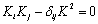is the limit case of dispersion relation of hot plasma.

Keywords: Dispersion Relation, Waves in Hot Plasma, Dielectric Tensor

1. Introduction

The dispersion relation and the dielectric tensor of waves in plasma have been extensively studied over the last decade ", , , , ". The primary purpose of these investigations is to gain a better understanding of plasma properties, specially studying waves propagation in plasma and producing renewable energy by the thermonuclear fusion controlled reaction. The subject of waves propagation in plasmas can not been considered as a simple problem, from mathematically point of view. In collisionless plasma it involves the use of Maxwell’s equations for the components of the electromagnetic field, coupled to a set of Vlasov equations for the distributions of each of the plasma species.

Macroscopic instabilities of plasmas are important subjects in fusion research and have been extensively studied by use of magnetohydrodynamics. In a high temperature plasma, however, kinetic effects play a vital role in stabilizing and destabilizing plasmas. In most of previous analyses, kinetic effects are involved perturbatively.

Dielectric tensor can be derived for different physics models such as multi-fluid model, kinetic model (without drift motion), drift kinetic model, drift kinetic model taking account of particle orbit, and so on. Propagation of electromagnetic waves in plasmas is the basis for understanding their optical properties in different ranges of frequencies and wavelengths. A large number of articles and books are now available, concerning derivation of dielectric tensor and dispersion relation for plasmas ", , , ".

In order to study the propagation of electromagnetic waves in plasmas, the dispersion relation of waves is the used fundamental tool because it connects two dependants variables of plasma: the angular frequency (Ω) and the propagation waves vector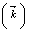as in expression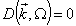where D denote the flux density of electric current.

The rotating electromagnetic field in which our hot plasma is located has been described by Lofo L.B. and Kazadi M.B. . The rotating field axe has been chosen along the OZ with angular velocity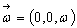This paper is devoted to derive the dispersion equation of waves for hot plasma located in a rotating electromagnetic field. To achieve our purpose, our work is subdivided as follow: in section 2, we derive the dielectric tensor of waves for hot plasma located in a rotating electromagnetic field . In section 3, we calculate the dispersion relation of waves for hot plasma and then, the section 4 will content our conclusions.

2. Derivation of Dielectric Tensor for Waves in Hot Plasma ", , , , , , , "

Let us consider our plasma with two species of particles, each denoted by the index "a". Each species is describe by a distribution function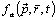, where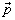,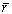and t are respectively momentum,  position vector and the time. This function is normalized as follows: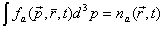(1)

Where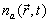is the number of particles of type "a" by unit of volume, at the position vector r and the time t. In the absence of collision the behaviour of the system is governed by the Vlasov - Maxwell system of equations.

A plasma is said to be hot when the aleatory component of particles velocity is not neglected i.e. one can take into account of temperature effects. In this approximation the distribution function of plasma particles will be given by the Maxwell-Boltzmann distribution function  as follow: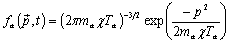(2)

where the suffix ²a² takes the two values of particles component (ions or electrons),

fa: distribution function of particle kind ²a²

p: particles moment

ma: particles mass of kind ²a²

c: Boltzmann constant

Ta: particles temperature of kind ²a²

The dielectric tensor of plasma is as follow: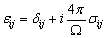(3)

where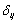is the delta Dirac distribution function and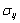is the electrical conductivity tensor of plasma. This last term is given by :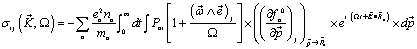(4)

where the:

ea: particles electrical charge of kind ²a²

na: particles electrical density of kind ²a²

Pai: particles moment of kind ²a²  and ith line

From the expression (3) the product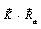can be written as follow:Remembering that: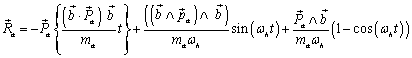(5)

and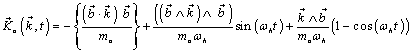(6)

Integrating overthe expression (4), we obtain the electrical conductivity tensor as follow: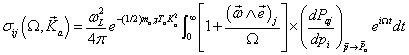(7)

where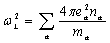is the Larmor frequency or the plasma frequency for the particles of species "a", and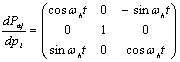is the Jacobian 

We can write the different components of electrical conductivity tensor of hot plasma in the form: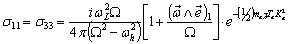(8)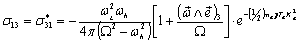(9)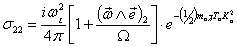(10)

Therefore(11)

Where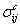is the electrical conductivity tensor of waves for hot plasma located in a rotating electromagnetic field .

From the expression (11), by putting it in the expression (2), we find the electrical dielectric tensor of hot plasma as follows:(12)

where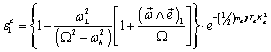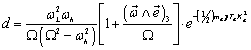where the super fix "c" stand for "hot".

One can see that the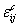components given by equation (12) are exactly equivalent to those appearing in Ref. , except that they content a temperature parameter in their exponential part.

3. Calculation of Dispersion Relation of Waves in Hot Plasma "[7, 8], , "

For the sake of simplicity we shall consider in this work that our plasma is a completely ionised gas i.e. it constituted of charged particles in electromagnetic fieldand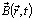. The laws of electromagnetic theory establish the connexion between the average values of the charge and current density on the one hand and the strength of the electrical and magnetic field in the other hand. These laws can be written in the form of the system of differential equations of Maxwell equations. The system of Maxwell equations can be written in the form :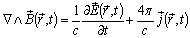(a)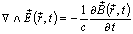(b)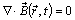(c)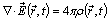(d)

where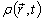et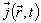are respectively the electrical charge and current density.

By combining the Maxwell equations (a) and (b) we obtain the following expression: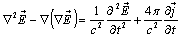(13)

The relation (13) is the wave’s electromagnetic propagation equation for hot plasma.

Applying to this expression (13) Fourier transforms , we obtain the following formula:(14)

where K is the intensity the wave vector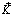and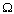the pulsation or angular frequency of plasma. Remembering that the electrical current density is connected to the electrical field by the expression: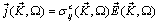(15)

By substituting in the expression (14) the expression (15) we obtain: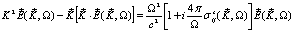(16)

Which is a system of ‘’n’’ equations to be solved. The component ‘i’ of this system of equations can be written: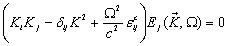(17)

From the solubility condition for this system of equations we find the dispersion relation: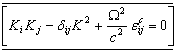(18)

In order to see how this dispersion relation varies with respect to the temperature, let us consider the non-zero components of. Indeed,

For i=j=1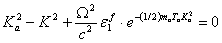(19)

And for i=j=2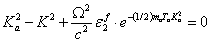(20)

The expression (18) of dispersion relation hot plasma has in its exponential part, the parameter ²temperature². Its presence indicates clearly that the temperature effects play an important capital role in the waves propagation in hot plasma located in rotating electromagnetic field. When thermal motion is taken into consideration the dielectric tensor is not Hermitean.

As the plasma considered in this work is hot, we observe that the dispersion relation depends on temperature parameter Ta. And, this parameter is an exponential factor in the expression of the dielectric tensor of cold plasma located in a rotating electromagnetic field. More the temperature is higher, more is the electrical conductivity of plasma (weak is the resistivity of hot plasma).We observe also that:

1) the temperature parameter Ta tends tozéro, the exponential factor tends to unity.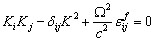which is the dispersion relation of cold plasma obtained in the previous work3.

2) the temperature parameter Ta tends to infinity when exponential factor tends to zero.which is the limit case of dispersion relation of hot plasma.

4. Conclusion

In this work we find that the dispersion relation has a temperature parameter in its exponential part. Consequently, this dispersion relation depends on three variables: wave’s vector, angular frequency i Ω and temperature parameter Ta of particles kind ‘‘a’’. As the considered plasma is hot, we observe that the dispersion relation depends on temperature parameter Ta. And, the temperature parameter is as exponential factor dielectric tensor of cold plasma located in a rotating electromagnetic field. More the temperature is higher, more the electrical conductivity of plasma is great (weak is the resistivity of hot plasma). We observe also that:

the temperature parameter Ta tends to zéro, the exponential factor tends to unity.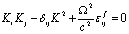which is the dispersion relation of cold plasma obtained in the previous works , .

the temperature parameter Ta tends to infinity when exponential factor tends to zero.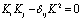which is the limit case of dispersion relation of hot plasma.

References

1. KAZADI M.B, LIYOKO .M and NYAMU M, Physica Scripta, 80, 2009).
2. Hui-Bin Qiu, Hai-Ying Song and Shi-Bing Liu, Physica Scripta, Vol. 90, N° 10, 1402 (2015).
3. Daniel Verscharen and Benjamin D. G. Chandran, Astrophys. J., vol. 764, N° 88, 1-12 (2013).
4. L.F. Ziebell and R.S. Schneider,BrazilianJournal of Physics, Vol. 34, N°3B, 1211-1223 (2004).
5. D. Verscharen et al.,The Astrophysical Journal, Vol. 773, N°8, 1-8 (2013).
6. Jun Zhu and Peiyong Ji, Plasma Phys Control. Fusion, Vol 54, N° 6 2012.
7. Roland H. T. and Nikolaos K. U., Radiowaves and Polaritons in Anisotropic Media,(WILEY-VCH Verlag GmbH & Co. KGaA, Weinheim, 2006).
8. Chen, F. F., Introduction to Plasma Physics, (New York: Plenum. 1974).
9. Akutsu T. and Fukuyama A., J. Plasma Fusion. SERIES.Vol. 6, 164-168 (2004).
10. S.S. Pavlov,Problems of Atomic Science And Technology, 2011. № 1.53Series: Plasma Physics (17), p. 53-55.
11. LOFO L.B. and KAZADI M.B., Physica Scripta, 54, 370 - 375, (1996).
12. KAZADI M.B and LOFO L.B., Physica Scripta, 58, 496-498, (1998).
13. Jean Louis D. et Abraham B, Physique des plasmas 1, savoir actuels Inter Editions / CNRS Editions, Paris, (France) 1994.
14. KAZADI M B, Sur le tenseur diélectrique du plasma dans un champ électromagnétique en rotation, Mémoire de Licence, Faculté des Sciences, Département Physique, Université de Kinshasa, (Kinshasa avril 1988), (inédit).
15. T. H. Stix, The theory of plasma waves, (AIP, New York, 1992).
16. Klimontovich, Yu. L., "The Statistical Theory of Non equilibrium processes in a Plasma", (Pergamon Press Ltd, London, 1967).

 Contents 1. 2. 3. 4.
Article ToolsAbstractPDF(185K)